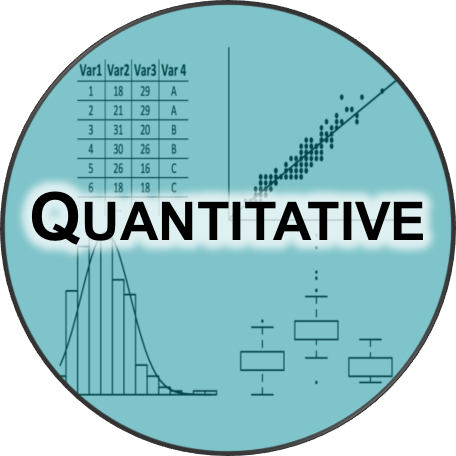# Comparing & Displaying Quantitative Data Assessment Test

10 Questions | Total Attempts: 129SettingsThe aim of quantitative data is to measure and quantify data, and generalize results from a sample. Take this assessment test to find out more.

• 1.
The following are features to focus on when comparing data sets except...
• A.

• B.

Center

• C.

Amount

• D.

Shape

• 2.
Where are dotplots positioned when they are used to compare data sets?
• A.

They are positioned, one above the other.

• B.

They are positioned, between each other.

• C.

They are positioned, side by side.

• D.

They are positioned, diagonally.

• 3.
Quantitative data is information that can be...
• A.

Measured and written down with numbers

• B.

Written and measured with alphabets

• C.

Measured but cannot be written down

• D.

Can be written down but not measured

• 4.
Which of the following is NOT a quantitative data?
• A.

• B.

The number of pennies in your pocket

• C.

• D.

The amount of water in the ocean

• 5.
The following are methods of displaying quantitative data except...
• A.

Dotplots

• B.

Center Plots

• C.

Line Graphs

• D.

Center charts

• 6.
The following are types of quantitative data except...
• A.

Measurements

• B.

Calculations

• C.

Estimates

• D.

Time

• 7.
Which of the following is a source of quantitative data?
• A.

Interviews

• B.

Primary data

• C.

Sources

• D.

Structured cards

• 8.
Secondary data consists of the following except...
• A.

Company reports

• B.

Dairies

• C.

Future events

• D.

Written accounts

• 9.
Which of these displays the distribution of a quantitative variable while preserving the individual values?
• A.

Stem and leaf

• B.

Tree

• C.

Branch

• D.

Tree diagram

• 10.
If observations cover a wide range, the spread is...
• A.

Smaller

• B.

Larger

• C.

Bit

• D.

Lower

Related TopicsBack to top